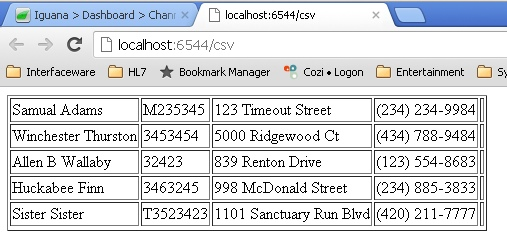# Display Excel csv file in HTML tables

Purpose:  I needed to convert excel spreedsheet into html tables at one point.  Here I have taken that concept and made a little project that you could begin with.  You could use the link below which discusses how to have Iguana convert an excel into a csv for you automatically.  Also you may choose to not have this a web service but to produce an actual html page. However, as a way to display this easily, I have chosen to display as a web service and I included a csv file inside the project

Steps to Build the Channel:

• Source = From https
your url path can be anything I chose ‘html’
example of web call http://localhost:6544/html
• Filter = None
• Destination = to QueueWriting the Code:

2. Save the milestone
3. Run the channel
4. Press on the link from the tool tip when hovering over your channel source

Review the Code:

```require 'split'

function trace(A) return end

-- Search whole directory = Directory = 'edit\\admin\\other\\Patient'

function main(Data)

queue.push{data=Data}
--more than one = local F = io.popen('cd '..Directory .. ' && dir *.csv /B')
local F = io.popen('cd '..Directory .. ' && dir Patient_List.csv /B')
--local F = io.popen(Directory)

local Files = R:split('\n')
trace(Files)
TT = ''

for i =1, #Files do
if Files[i] and Files[i] ~= '' then
trace(Files[i])
TT = TT..'\r'..PullCSV(Directory..Files[i])
trace(TT)
end
end

net.http.respond{body=TT}

end

function PullCSV(F)
local F = io.open(F)
if F then
local TableComplete = build(L)
return TableComplete
end
end

function build(csv)
if csv ~= nil and csv ~= '' then
local L = csv:split('\n')
local newT = {}
--start html table
newT = "<TABLE border ='1'><TR>"
--create inside of html table
for i =2, #L do
newT[i] = "<TD>"..L[i].."</TD></TR><TR>"
newT[i] = newT[i]:gsub(',', '</TD><TD>')
end
newT[#L+1] = "</TR></TABLE>"
--put it all together and return
Thtml = ''
for k,v in pairs(newT) do
Thtml = Thtml..v
end
return Thtml
end
end```

End Result

• You can make any adjustment to the HTML that you would like so that the formatting looks nicer but below is an example of the result.